Lei, J., Lei, D., Wang, S., and Liu, Z. (2023). "Stress relaxation characteristics of crushed cane tail straw," BioResources 18(1), 143-160.

#### Abstract

To gain insight into the mechanical properties of crushed sugarcane tail leaves during stress relaxation, a self-made compression equipment was used in this study. The variation law of different factors on the stress relaxation process of crushed cane tail was explored and a stress relaxation model was established. The three-element and five-element generalized Maxwell models were selected to fit the regression analysis of the stress relaxation curve of the crushed cane tail. The comparison showed that the determination coefficient R2 of the five-element stress relaxation model was higher, and a three-factor and three-level response surface test was designed. Following the quadratic regression polynomial of the stress rapid decay time and the equilibrium elastic modulus, the final optimization results obtained are as follows: The moisture content was 60.8%, the crushing particle size was 45 mm, the feeding amount was 150 g, and the stress rapid decay time was 14.0 s. The equilibrium elastic modulus was 129 kPa.

Stress Relaxation Characteristics of Crushed Cane Tail Straw

Junle Lei,* Dingyuan Lei, Shuaiwei Wang, and Zhaochong Liu

To gain insight into the mechanical properties of crushed sugarcane tail leaves during stress relaxation, a self-made compression equipment was used in this study. The variation law of different factors on the stress relaxation process of crushed cane tail was explored and a stress relaxation model was established. The three-element and five-element generalized Maxwell models were selected to fit the regression analysis of the stress relaxation curve of the crushed cane tail. The comparison showed that the determination coefficient R2 of the five-element stress relaxation model was higher, and a three-factor and three-level response surface test was designed. Following the quadratic regression polynomial of the stress rapid decay time and the equilibrium elastic modulus, the final optimization results obtained are as follows: The moisture content was 60.8%, the crushing particle size was 45 mm, the feeding amount was 150 g, and the stress rapid decay time was 14.0 s. The equilibrium elastic modulus was 129 kPa.

DOI: 10.15376/biores.18.1.143-160

Keywords: Crushed cane tail; Stress relaxation; Generalized Maxwell model; Equilibrium elastic modulus; Rapid stress decay time

Contact information: College of Mechanical and Control Engineering, Guilin University of Technology, Guilin, 541004, P.R. China; *Corresponding author: 40911409@qq.com

INTRODUCTION

Sugarcane is an important low-cost economic crop. It is rich in carbohydrates, sucrose, and inorganic elements. In 2021, the sown area of sugarcane in China was 1,353 thousand hectares, of which Guangxi’s sown area accounted for about 65% of the country’s total. China’s sugarcane imports in 2021 were 1.4067 million tons, Exports were 0.06 million tons. However, the utilization rate of such a large amount of cane tail is less than 10% in China (Li et al. 2015; Tian 2020). At present, the use of sugarcane tail is mainly for silage. Silage technology is used to shorten the feeding cycle of cattle and sheep in winter and reduce the pressure on farmers. Because the stem of the cane tail is thick and hard, it is difficult to compact after cutting, and this can easily cause silage failure. The silage produced by it is more convenient for storage and transportation after being sealed and packaged. To strengthen the silage effect, it must involve the compression, transportation, baling, and other problems in the process of sugarcane silage.

Up to now, a large number of scholars at home and abroad have done research on the stress relaxation test of some crops. Kugler et al. (1969) first proposed a straw compression rheological model. Followed by this, the generalized Maxwell model was used to analyze the stress relaxation rheological properties during straw compression, and the rheological equation was deduced (Mohsenin and Zaske 1976; Bock et al. 1989). Peleg (1983) used a nonlinear visco-plastic solid material-based rheological model to describe the relaxation characteristics of agricultural fiber materials during the compression process, and obtained the expression of relaxation modulus. Wang et al. (2017) expounded the stress relaxation behavior of rice based on the time, moisture content, and temperature. Lastly, they described the relationship curve using the theory of thermo-rheological materials and the establishment of a 7-element generalized Maxwell model. Chen et al. (2013) recognized a fractional constitutive model for the stress relaxation behavior of wheat straw, which clearly described the fast and slow relaxation stages. Du et al. (2018) established a stress relaxation model for sweet sorghum straw with different moisture content, compression density, length of chopped segment, and compression speed, and analyzed the relationship between the four factors. Waananen and Okos (1992), Chen et al. (2019), Li et al. (2013), Shi et al. (2017), Yuan et al. (2012), Sheng et al. (2014), Wang et al. (2021), Abedi and Takhar (2022), and Xiao et al. (2020), respectively analyzed corn kernels, barley straw, industrial hemp fiber, wormwood straw, wheat stalks, corn kernels, machine-harvested seed cotton, banana, and rice seedlings. Caicedo et al. (2021) established a viscoelastic plastic model for the compressive properties of sugarcane residues. Zhang et al. (2021) used the Maxwell model to study the mechanical properties of cabbage under lateral and longitudinal loadings and analyzed the relaxation characteristics in different compression directions. Lei et al. (2015) studied the stress relaxation characteristics of rice straw, established a three-element generalized Maxwell model, and optimized rice straw moisture content, bale dry matter quality, rolling steel roll speed, and rice straw feeding amount. Solomon et al. (2002) used axial and radial compression tests to study the creep and stress relaxation models of potato tissue cylindrical samples at different temperatures. Yinyan et al. (2017) conducted a compression experiment of Artemisia selengensis straw with different soil moisture, diameters, and directions. In particular, these results showed that the compressive strength and elastic modulus decreased with the height of stalk position, while the deformation increased. In addition, with the increase in the stalk diameter, the bending strength and fracture mechanical work increased, while the deformation decreased.

As shown above, there have been many reports on crushed wheat, rice, corn, and other crops, but the research on the mechanical properties of the stress relaxation of crushed sugarcane tail leaves rarely has been reported. Therefore, the stress relaxation test of crushed cane tail was conducted in this paper, and the significant influence of single factor and multiple factors was analyzed. Finally, through the Box-Behnken 3 factors and 3 levels analysis, with the rapid stress decay time and equilibrium elastic modulus as the evaluation criteria, a quadratic regression equation was established, and the optimal parameter combination was obtained. These results provide important physical parameters and a theoretical research basis for the optimization of sugarcane collection machines and silage in the future.

EXPERIMENTAL

Materials

The WDW-100 type micro-controlled electronic universal testing machine (Jinan Zhongbiao Instrument Equipment Co., Ltd., Jinan, China) was utilized, as shown in Fig. 1. It has three control modes of stress, strain, and displacement, which can be switched randomly between control modes, and automatically collect and process test data, draw various curves, and print test reports. The compression link used was 200 mm. The inside of the compression cylinder was cleaned and made rust-proof and dust-proof. The friction force was as far to avoid the impact of impurities on the test as possible to avoid the influence of the test error on the data. There were different regular exhaust cylinders with a diameter of 5 mm at the bottom and wall of the compression cylinder, which was convenient for the discharge of crushed sugarcane tail juice and air during the compression process to facilitate cleaning of residues. Auxiliary equipment included electronic balances, electronic scales, measuring cups, and Vernier calipers.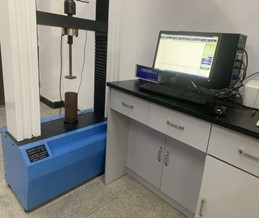Fig. 1. Compression test device

Test Sample

In this study, the sugarcane root from cane field planting base of Guangxi Academy of Agricultural Sciences (Gui Lin, China) was used, as shown in Fig. 2. The test area was 10 mu, 2 rows of sugarcane were planted between the rows, the row spacing was kept at 30 cm, the plant spacing was 25 cm, 3000 plants were planted per 667 m2, the seeding amount was approximately 60 kg, and the cover of about 10 cm of soil was crushed, leveled, and covered with film. The sugarcane variety Guitang No. 42 was used as the raw material for the test. The healthy, disease-free, and no pests crushed cane tail straw was collected with a sickle and brought back to the laboratory, where it was placed in a dry and easily ventilated environment for subsequent treatment. The cane tail was added into the crushing device, and the slip screen was used to screen-out the smaller particles less than 15 mm, 25 mm, and 45 mm respectively. Because the collected sugarcane tails contained sugarcane leaves, tail stems, and other impurities (including weeds and bad leaves), the crushed sugarcane tails were randomly selected from the sample group for 3 groups. The proportion was 51.8%, and the average proportion of impurities was 3.2%.Fig. 2. Test sample

Experimental Design and Methods

Stress relaxation analysis using various feeding amounts

Approximately 150, 200, and 250 g of test sample was added into the compression cylinder. The procedure was to first make a set of test samples with a feeding amount of 150 g, and then make test samples of 200 g and 250 g in turn. The moisture content, crushing particle size, and compression density were set to the same level. Compression was stopped when the target compression density reached 500 kg/m3. The crushed cane tails were then held for 650 s. At this time, the material compressed and molded by the crushed cane tail undergoes stress relaxation in the compression cylinder. The experiment was repeated 3 times, and the final results were averaged. The test data was first organized into an Excel table, and the relationship curve of stress and time for different feeding amounts was obtained. Finally, the data was imported into Origin2020 (Microcal, 2020, Northampton, MA, USA) for curve fitting, and obtained the stress relaxation model.

Stress relaxation analysis at various moisture content

Approximately 20%, 50%, and 80% of crushed cane tails were used for this purpose, and the feeding amount, crushing particle size, and compression density were set to the same level. Compression was stopped when the target compression density of 500 kg/m3 was obtained. The simulations of the two models for various water contents were compared.

Stress relaxation analysis of different crushing particle sizes

The crushed cane tail samples smaller than 15, 25, and 45 mm sizes were used, and the feeding amount, moisture content, and compression density were kept at the same level. When the target compression density of 500 kg/m3 was obtained, the compression was stopped. In the same way as the above method, the stress relaxation models of different crushing particle sizes under the two models was then compared.

Stress relaxation analysis of different compression rates

The moisture content, feed amount, and crushing particle size were set to the same level, and the compression rates of 20 mm·min-1, 50 mm·min-1, and 80 mm·min-1 were targeted as the compressive stop behavior. The stress relaxation process at 3 compressive densities was recorded and the respective stress relaxation model at 3 compressive densities was analyzed in the same way.

Stress Relaxation Model Selection

The stress relaxation model of agricultural straw was used as the generalized Maxwell model. Therefore, this study used Origin 2020 software to establish a generalized Maxwell model with a different number of units to fit and a regression analysis of the stress relaxation curve of the crushed cane tail was obtained. The model expression is shown in Eq. 1,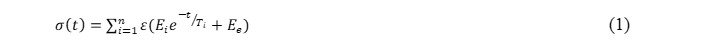where σ(t) is the stress value corresponding to any time (mm/s), is the initial strain, Ti is the stress relaxation time (s), e is a numerical constant, approximately equal to 2.718, Ei is the relaxation elastic modulus (kPa), Ee is the equilibrium elastic modulus (kPa).

The generalized Maxwell model consists of one or more Maxwell models connected in parallel with elastic elements. The number of model parameters depends on the number of viscous elements (sticky pots) and elastic elements (springs). The model diagram is shown in Fig. 3.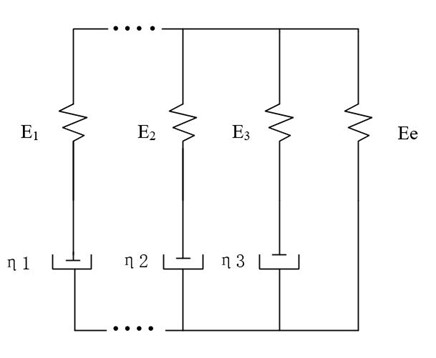Fig. 3. Generalized Maxwell Model

Test Index

A generalized Maxwell model is established based on the rheological properties of solids in agricultural materials science. The decay is the initial strain, Ti is the stress relaxation time, Ee is the equilibrium elastic modulus, and Ei is the relaxation modulus that can be used to describe the material relaxation behavior and viscoelastic deformation behavior. Therefore, it can be used as an indicator for analyzing the stress relaxation characteristics of crushed cane tails.

TEST AND ANALYSIS

Effect of Water Content on Stress Relaxation Properties of Crushed Cane Tail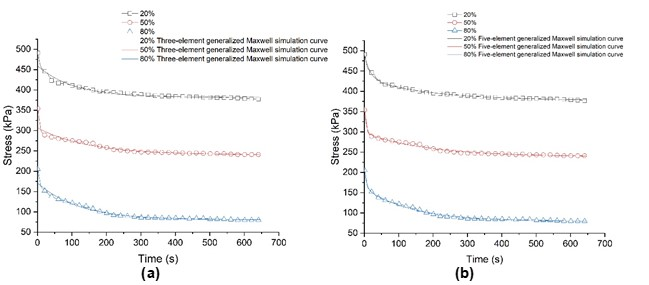Fig. 4. (a) Three-element generalized Maxwell model of water content; (b) Five-element generalized Maxwell model of water content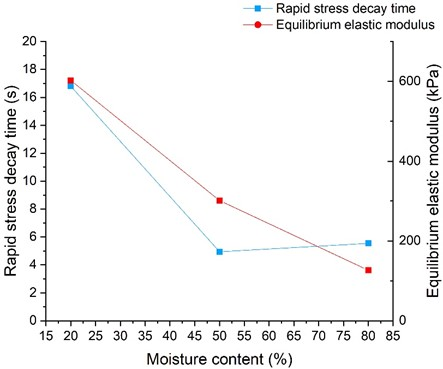Fig. 5. Moisture content- Rapid stress decay time-Equilibrium elastic modulus relationship curve

Figure 4(a) represents stress versus time plots for 20%, 50%, and 80% moisture content in that order and three-element generalized Maxwell model curve of water content; Figure 4(b) represents stress versus time plots for 20%, 50%, and 80% moisture content in that order and five-element generalized Maxwell model curve of water content and the fitting of each water content with the three-element generalized Maxwell model and the five-element generalized Maxwell model. It can be seen from Fig. 4 that with the increase of water content, the maximum stress value of the crushed cane tail when it was compressed to 500 kg/m3 density in the compression cylinder was smaller. When the moisture content was low, the stress of the crushed cane tail changed greatly. The stress gradually decreased and leveled off with time. In Fig. 4(a), the three-element generalized Maxwell fitting curve and the experimental data did not completely fit, while the fitting effect of the five-element generalized Maxwell curve in Fig. 4(b) was better. It can be seen from Fig. 5 that with the increase of water content, the equilibrium elastic modulus of the crushed cane tail gradually decreased; that is, the stress decay time and elastic recovery force were also smaller during the stress relaxation process. This phenomenon shows that the stress decay time can be reduced with high water content.

Effect of Crushing Particle Size on Stress Relaxation Characteristics of Cane Tail

Figures 6(a) and (b) respectively show the relationship between stress and time when the crushing particle size was less than 15 mm, 25 mm, and 45 mm, and the simulation of each crushing particle size with the three-element and the five-element generalized Maxwell models. It can be seen from Fig. 6 that with the increase of the crushing particle size, the maximum stress value also increased gradually. When the crushed cane tail was under stress relaxation from the crushed particle size of 45 mm, the stress suddenly dropped sharply, so it can be seen from Fig. 6(a) that the three-element generalized Maxwell model cannot completely simulate the complete change of the stress relaxation curve during the pressure holding process. The fitting effect of the five-element generalized Maxwell model in Fig. 6(b) was better. Figure 7 shows that with the increase of crushing particle size, the stress decay time decreased slowly, and the equilibrium elastic modulus increased rapidly at first and then increased slowly. It shows that when the crushing particle size increased, the gap of the material in the compression cylinder became larger, and the larger the equilibrium elastic modulus is, the final molding effect of the material became worse.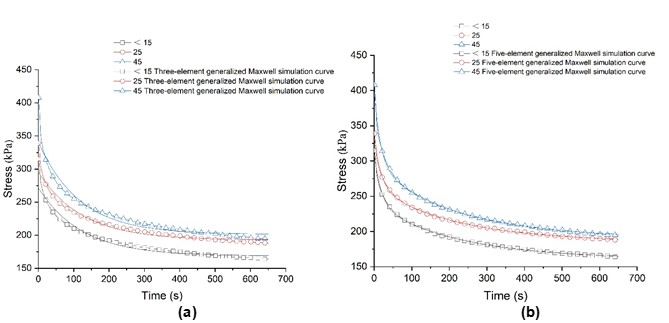Fig. 6. (a) Three-element generalized Maxwell model; and (b) Five-element generalized Maxwell model for crushing particle size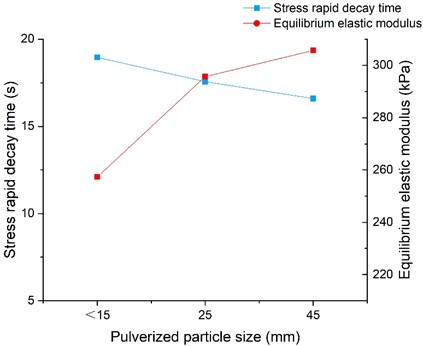Fig. 7. Pulverized particle size-Stress rapid decay time-Equilibrium elastic modulus relationship curve

Effects of Feed Amount on Stress Relaxation Characteristics of Crushed Cane Tail

Figures 8(a) and (b) respectively show the relationship between stress and time of the feeding amount of 150, 200, and 250 g, and each feeding amount is simulated with the three-element and the five-element generalized Maxwell models. It can be seen from Fig. 8 that with the increase of the feeding amount, the maximum stress value was basically the same, and the feeding amount had no obvious effect on the internal friction and extrusion force of the crushed cane tail during the stress relaxation process. From Fig. 8(b), it can be seen that the five-element model fit better. It can be seen from Fig. 9 that with the increase of the feeding amount, the rapid stress decay time gradually decreased, and the equilibrium elastic modulus decreased rapidly at first and then slowly decreased. It shows that when the feeding amount of crushed cane tail was a certain amount, it reduced the rapid decay time of stress and the equilibrium elastic modulus.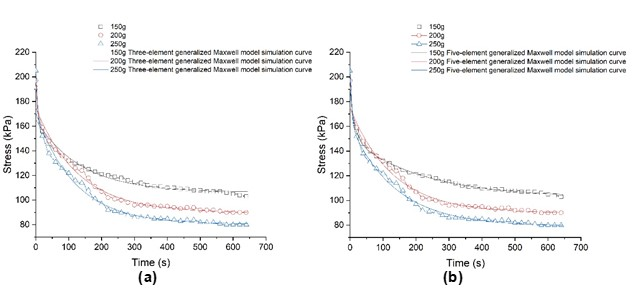Fig. 8. (a) Three-element generalized Maxwell model; and (b) Five-element generalized Maxwell model for various Feed amounts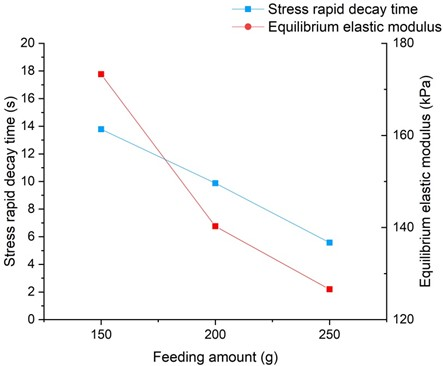Fig. 9. Feeding amount-Stress rapid decay time-Equilibrium elastic modulus relationship curve

Effect of Compression Rate on Stress Relaxation Properties of Crushed Cane Tail

Figures 10(a) and (b) show the stress-time relationship curves of compression rates of 20, 50, and 80 mm·min-1, respectively, as well as the relationship between the compression rates, and the three-element and the five-element generalized Maxwell models. It can be seen from Fig. 10 that with the increase of the compression rate, the maximum stress value was basically the same, that is, the compression rate had little effect on the stress relaxation process. The fitting effect of the five-element generalized Maxwell model reflected from Fig. 10(b) was better. As shown in Fig. 11, the time range of rapid stress decay was approximately 17 to 21 s, and the range of equilibrium elastic modulus was about 250 to 300 kPa, so the compression rate had no obvious effect on the two.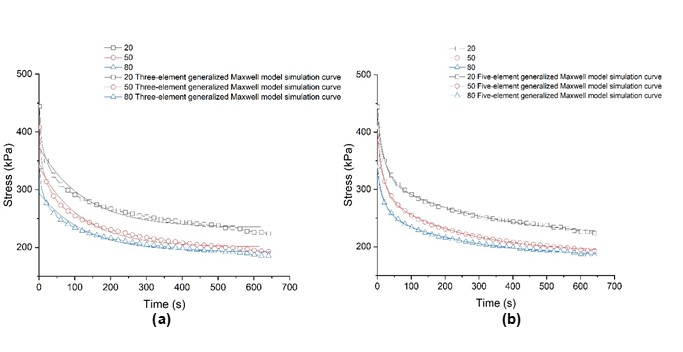Fig. 10. (a) Three-element generalized Maxwell model for Compression rate; (b) Five-element generalized Maxwell model for compression rate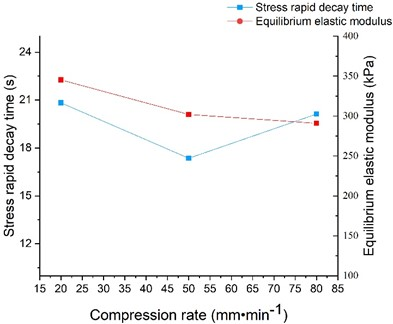Fig. 11. Compression rate-Stress rapid decay time- equilibrium elastic modulus relationship curve

The parameters of each factor simulation regression model are shown in Tables 1 and 2.

Table 1. Three-Element Generalized Maxwell Model Fitting Parameters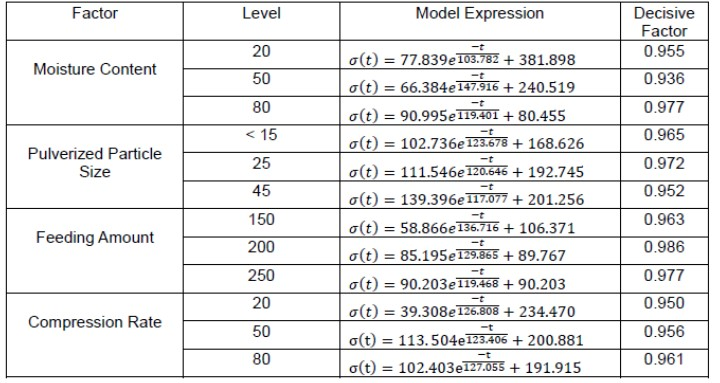Table 2. Five-Element Generalized Maxwell Model Fitting Parameters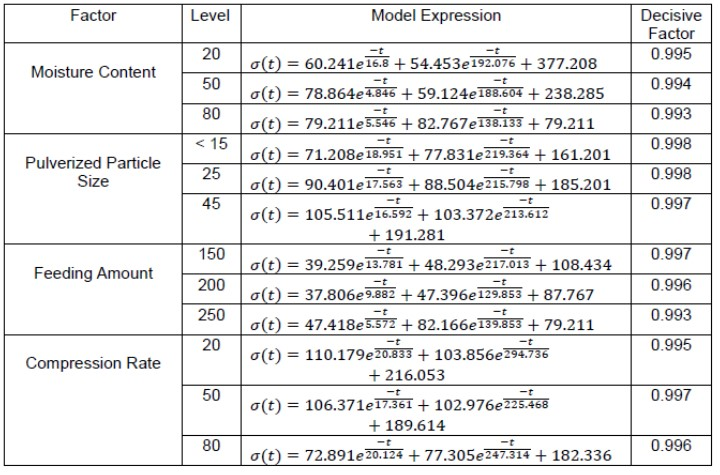From Tables 1 and 2, it can be seen that the five-element generalized Maxwell model had a higher determination coefficient than the three-element generalized Maxwell model, and the determination coefficient R2 ≥ 0.993, which is reflected in Figs. 3 through 10. For example, it can be seen from Table 1 that the determination coefficient of 150 g feeding was 0.9634. The actual determination coefficient was 0.96303, and the adjusted determination coefficient was 0.96292. In order to get a more accurate determination coefficient, a Five-Element Generalized Maxwell model was prepared. As can be seen from Table 2, the determination coefficient was very close to 1. The actual determination coefficient and adjusted determination coefficient of 3 and 5 elements in Tables 3 and 4. It can be seen that, based on the adjusted R2 values, the Five-Element Generalized Maxwell model is more suitable for the actual situation of crushed cane tail.

Table 3. Three-Element Generalized Maxwell Model Fitting Parameters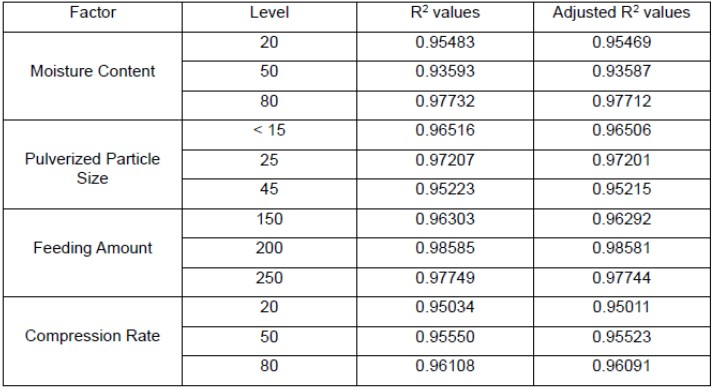Table 4. Five-Element Generalized Maxwell Model Fitting Parameters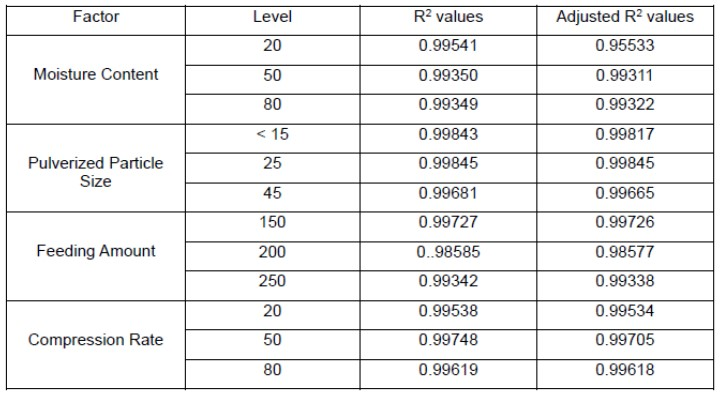According to the findings of this article, it can be concluded that the compression rate had no obvious effect on the rapid stress decay time and the equilibrium elastic modulus, so the moisture content (A), crushing particle size (B), and feeding amount (C) were selected as the test factors, and the Box-Behnken experimental design was used. Three-factor and three-level response surface analysis tests were carried out. Each group of tests was repeated three times, and the test results were averaged. The levels of each test factor are shown in Table 5. During the stress relaxation process of crushed cane tail, the rapid stress decay time and equilibrium elastic modulus were selected as evaluation indicators.

Table 5. Coding Table for Stress Relaxation Test of Crushed Sugarcane Tail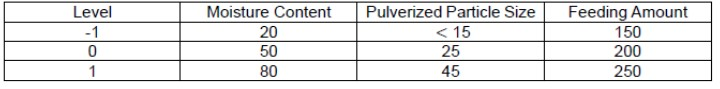Stress-relaxation Regression Model and Analysis of Variance for Crushed Cane Tail

The test results were obtained according to the appeal test plan, and the analysis was imported into the Design-Expert 8.0 (Stat-Ease, Minneapolis, MN, USA) software, and the variance analysis table of the rapid decay time of the crushed cane tail stress and the equilibrium elastic modulus were obtained, as shown in Tables 6 and 7.

Table 6. Analysis of Variance of Rapid Stress Decay Time in Crushed Cane Tail Stress Relaxation Test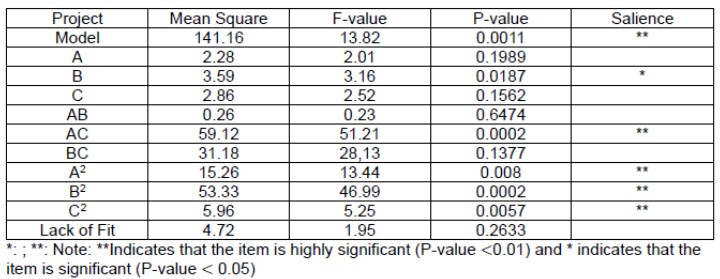The lack of fit item in the table was not significant (P-value = 0.26330.05), that is, the regression model was significant (P-value = 0.0011 0.05), indicating that the quadratic regression model was feasible. The quadratic regression model of the rapid stress decay time of the crushed cane tail was: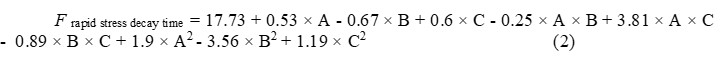Table 7. Analysis of Variance Table of Equilibrium Elastic Modulus for Crushed Cane Tail Stress Relaxation Test